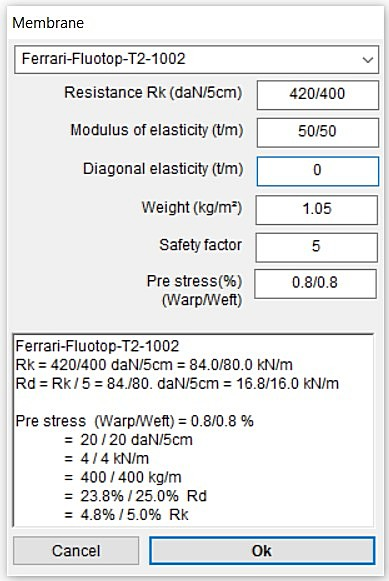##### Basic concepts

The membrane structures are constructed by providing a pretensioning force to membrane. The reasons for the prestressing are several:

1. Self weight of the membrane would deform it, forming unforeseeable wrinkles and making her exposed to uncontrolled movements.
2. Considering that the membranes can only withstand tension, if we put it a tensioned state, when there are loads produced by external actions (snow, wind,…) to create compression, this compression will be compensated by the prestressed tension and, probably, the membrane will remain in tension.
3. Iterative calculation of this type of structures will be much faster due to the convergence of the calculation is greater in the tensioned state.
4. Finally, the deformation of the membrane facing external actions will be much lower.

In point 2, however, we need to make a clarification. It is possible that the membrane is subject to loads of tension due to external actions. In this case the pre-stressing will will only increase the tension loads, so the resistance of the membrane will be compromised sooner than expected.

This is why you must use a pretension loads that do not compromise the resistance of the membrane. In this sense, “European Design Guide for Tensile Surface Structures” recommended values of prestress not less than 2.5 % and no greater than 6% of maximum tensile strength of the membrane. If we bear in mind that a typical value of factor of safety of the membrane is 5, we see that these values translate into 12.5 and 30% of the resistance capacity of membrane.

##### WinTess3 and prestress

The program allows you to set the prestress of the membrane in different menus or paragraphs, although always lead us to the same window.We can find the window of prestress in different ways:

• Using the menu Membrane | Edit
• Menu Calculate | Surface loads that we will open the window of the surface loads. In this menu, we can modify the prestress in the bottom right, . If we try to do this, it will open the edit window of membranes.
• Menu Patterns | Draw patterns. In this case, the calculation has already been done and we are about to draw the patterns of the membrane. It is clear that we must compensate mechanically for those patterns. This means that we must make them smaller, so that when installed on site it should grow (gets tension) to take its final position.
The program reminds us the prestress used in the calculation but it allows us modify it, as there may be other reasons for compensation.
In any way, we should be very careful if we modify the prestress in this stage, because it weakens the calculation already done.

As an example, a membrane type, Ferrari-Fluotop-T2-1002, is shown on the right.

A pretension of 0.8% is applied in both directions of the warp and the weft. (If these two values are equal, we can enter only one number: 0.8 /0.8 = 0.8 ).

In the lower text box we see the results of this pretension. For a membrane with a modulus of elasticity of 50 t/m (500 kN/m), a pretension of 0.8% (0,008 ) is equivalent to a stress of:

`σ = ε x E  = 0.008 x 500 = 4 kN/m`

The program gives us this value in several units, daN/5cm, kN/m, kg/m for convenience and then relative values with respect to the maximum tensile stress and useful resistance affected by the safety coefficient.